Solving Rational/Fractional Equations

Rational (or fractional) equations can be solved by:
1.  determining the least common denominator (LCD) for the problem,
2.  multiplying both sides of the equation by the LCD (multiply ALL terms),
3.  solving the resulting equation, and
4.  checking for possible
extraneous roots since the derived equation may not be equivalent to the original equation.

The graphing calculator is a wonderful back-up checking tool to solving such equations.  It is especially helpful in determining extraneous roots.  Note the examples below.

Note: Two possible graphing screens are being shown. The screens on the right are screens from an older calculator. If you have an older model (with an operating system prior to V2.40), that plots vertical lines ("heart beats") where the function is undefined, you will want to use DOT mode in these rational function problems to prevent these vertical lines from appearing.

Example 1:  Solve the following equation algebraically and check.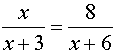Algebraic Solution: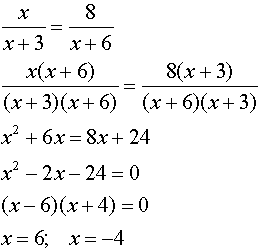Graphical check:
Enter the left side of the equation into Y1.
Enter the right side of the equation into Y2.
Be careful to include the parentheses for the denominators in this problem.
Use the INTERSECT option (2nd Trace #5).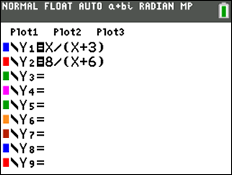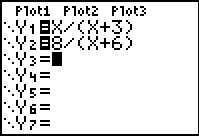In dot mode, the graph looks like: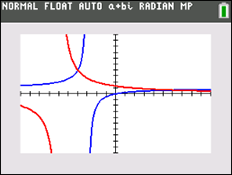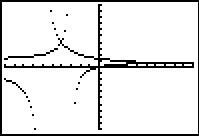Check: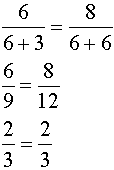Check: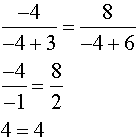Checking the two intersection points, gives: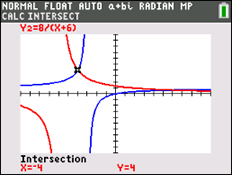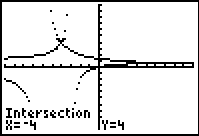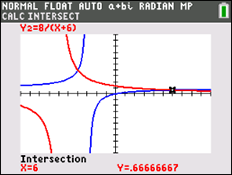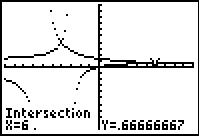Both roots check in this problem.

Notice that the graph shows TWO intersection points.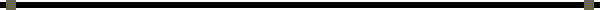Example 2:  Solve the following equation algebraically and check.Algebraic Solution: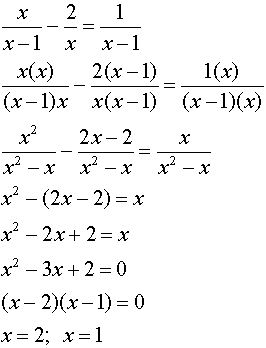Graphical check: Enter the left side of the equation into Y1. Enter the right side of the equation into Y2. Be careful to include the parentheses for the denominators in this problem. Use the INTERSECT option (2nd Trace #5).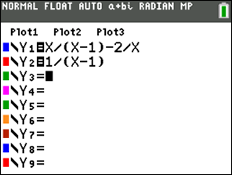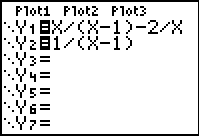In dot mode and standard window, the graph looks like: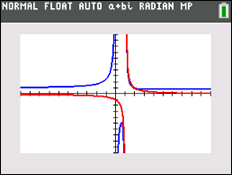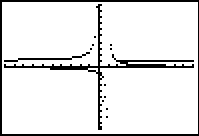Check: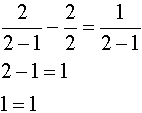Check: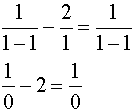Division by zero is undefined.  x = 1 is NOT an answer. x = 1 is an extraneous root. Zoom in and find the intersection: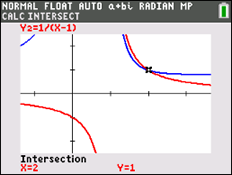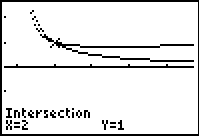You can move the spider near x = 1 and zoom in to look for an intersection.  There is NO intersection at x = 1.

NOTE:  When working with rational equations, it may be difficult to "see" the intersection point if the viewing window is a small representation of the graph.  You may want to enlarge the viewing window by adjusting the WINDOW settings or by using ZOOM (#2 Zoom In).  Remember, when using Zoom In, hitting ENTER the first time only registers the function.  You must hit ENTER a second time to activate the Zoom In option.  You can quickly return to the 10 x 10 viewing window by pressing ZOOM (#6 ZStandard).

Remember:  In Example 2, you can see that there is only one answer to the equation since there is only one point of intersection.  Should there be NO points of intersection, the answer will be the empty set.